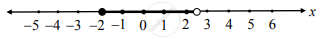# Sketch the graph which represents the solution set for the following inequation.– 2 ≤ x < 2.5 - Mathematics and Statistics

Graph

Sketch the graph which represents the solution set for the following inequation.
– 2 ≤ x < 2.5

#### Solution

– 2 ≤ x < 2.5
Here, x takes all values between –2 and 2.5 including –2.
∴ Solution set represents the bounded (semi-left closed) interval (–2, 2.5)
∴ the required graph of the solution set is as follows.Concept: Graphical Representation of Solution of Linear Inequality in One Variable
Is there an error in this question or solution?

#### APPEARS IN

Balbharati Mathematics and Statistics 2 (Commerce) 11th Standard HSC Maharashtra State Board
Chapter 8 Linear Inequations
Exercise 8.1 | Q 3. (vi) | Page 116